﻿
Reach Us+44-1522-440391
Concentration, Wavelength and Temperature Dependent Refractive Index of Sugar Solutions and Methods of Determination Contents of Sugar in Soft Drink Beverages using Laser Lights

# Journal of Lasers, Optics & PhotonicsOpen Access

All submissions of the EM system will be redirected to Online Manuscript Submission System. Authors are requested to submit articles directly to Online Manuscript Submission System of respective journal.

# Concentration, Wavelength and Temperature Dependent Refractive Index of Sugar Solutions and Methods of Determination Contents of Sugar in Soft Drink Beverages using Laser Lights

Belay A* and Assefa G
Applied Physics Program, School of Applied Natural Science, Adama Science and Technology University, Ethiopia
*Corresponding Author: Belay A, Applied Physics Program, School of Applied Natural Science, Adama Science and Technology University, P.O.Box 1888, Ethiopia, Tel: +251 22 111 0400, Email: [email protected]

Received Date: Jun 14, 2018 / Accepted Date: Jun 27, 2018 / Published Date: Jul 04, 2018

Keywords: Laser light; Refractive index; Temperature; Sugar solution and Soft drinks

#### Introduction

The refractive index n is a basic optical property of materials and its accurate value is often needed in many branches of physics and chemistry and has also several applications in many industries and materials to know the clarity of glasses and solid plastics [1-6]. By measuring the refractive index of a binary solution, one can determine the composition of the solution [3,4]. So, measurements of refractive index are widely used in many industrial and research applications to determine the concentration of the solution [3-6].

There are numerous methods for measuring the refractive index of a liquid solution that are well documented in literatures [1-10]. The diversity of methods is largely due to differences in applications, test objects and the required measurement accuracy. A separate class of devices for measuring the refractive index of transparent liquids consists of systems designed for examining liquids in cuvettes made of glass, quartz or other transparent materials. During the last decade, numerous practical devices based on the principle described above using various cuvettes have been constructed for determining the refractive index [6-9].

The refractive index varies with concentration, temperature, pressure, and wavelength [2-7,10,11]. Recent studies [2-4] provided the detailed study on the concentration mapping by the measurement of refractive index of liquids. Temperature coefficient of refractive index can also be used to calculate thermal expansion coefficient [2,4]. Several techniques are reported in literature for the measurement of concentration and temperature dependence of refractive index of sugar solution using various techniques [2-7]. Various techniques have been developed to determine the contents of sugar in solutions [12-14]. The techniques are Inter digital Capacitor Sensor to determine the contents of sugar in solution ; optical Coherence Tomography to determine blood glucose concentration in diabetic patients ; Quantification of sugar in soft drinks and fruit juices by Density, refractometry, infrared spectroscopy and statistical methods [14-16] are some of the techniques so far developed.

The above mentioned techniques are industrial made instrument used to determine the concentration of sugar in solution. Such kind of instrument is very expensive and also not available in most laboratories. So, in this research we used relatively inexpensive and locally constructed equilateral hallow prism and very sensitive laser light sources (Red and Green Diode, He-Ne) to determine the concentration, temperature and wavelength dependent refractive index of sugar solution and its contents in different soft drink beverages (Pepsi, Mirinda, Coca, Sprite, 7up and Fanta) using Snell’s law. An empirical expression was also derived for the wavelength dependent refractive index of sugar solution by fitting Cauchy’s equation to the experimental data using non-linear curve at the minimum discrepancy.

#### Materials and Methods

Materials

Locally constructed equilateral hollow prism, Red and Green Diode laser, He-Ne laser as light sources, digital electronic balance with a good accuracy for mass measurement were used. In addition, different apparatus such as measuring cylinder to measure the volume of water, mercury thermometer to control the temperatures of the solutions and magnetic stirrer with hot plate for heating and mixing the solution. In addition, deionized water as solvent, locally produced sugar and soft drink beverages (Pepsi, Coca, 7up, Sprite, Mirind and Fanta) were used as our sample.

Methods of measuring concentration dependent refractive index of sugar solutions

To measure the refractive index of different sugar solution standard samples of varying concentration of sugar (m/m: 5%, 10%, 15%, 20%, 25%, 30%, 35%, 40%, 45%, 50%) were prepared by dissolving the sugar in deionized water. For example 5% of sugar solution means dissolving 5 g of sugar within 100 ml of deionized water. Calibration curve developed from the graph of refractive index versus the concentration of the solution. Numerical procedure of fitting the experimental data was carried out by linear curve fitting using origin 8 software.

A theory and method for derivation of refractive index have been discussed elsewhere [1-2,5]. The angle of incidence is responsible for deviation produced in the path of light beam. The angle of deviation δ is minimum when incident and refracted angle of the prism are equal . Therefore, the refractive index, n of the material filled inside the prism or solid prism of apex angle (α) can be calculated using eqn. (1)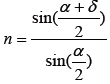(1)

Figure 1 indicated the experimental setup of the measurements. A monochromatic source of light (Red, Green Diode laser and He-Ne laser) was allowed to fall at angle of incident on the face of the liquid prism and the angle of minimum deviation was determined for each laser light. During the measurement the solutions were filtered before pouring into the hollow prism. The hollow prism was also rinsed carefully after every measurement. Each measurement has taken three times.

Figure 1: Experimental set up for the measurements of angle of minimum deviation using prism spectrometer.

Wavelength dependent refractive Index of sugar solution

An empirical relationship between the refractive index and wavelength of light for particular transparent materials can be expressed using Cauchy’s equation eqn. (2)(2)

Where n is the wavelength dependent refractive index, λ is the wavelength and A, B, C are the coefficients that can be determined for a materials. The fitting parameters are obtained by fitting Cauchy’s equation to the experimental data by non-linear curve flitting using origin 8.6 software.

Methods of measuring temperature dependent refractive

The temperature dependent refractive indices of the solution measured at the temperatures (308.15-343.15 K) inside hallow prism. The solutions were heated using magnetic stirrer with hot plate and its temperature controlled by placing the thermometer inside a solution. The angle of minimum deviation was measured at the interval of 5 K while the solution is cooling down from highest to lowest temperature. The temperature dependent refractive index measured for different mass fraction (0%, 10%, 20% 30%, 40% and 50%). Calibration curve developed from the graph of refractive index versus temperature of the solution.

Soft drink beverage solutions preparation

In order to measure the sugar contents in soft drink beverages (Pepsi, Coca, 7up, Sprite, Fanta and Mirinda) each sample was poured into a beaker and stirred for 5-10 minutes by magnetic stirrer to remove gases within the samples. The refractive index of each solution was calculated from angle of minimum deviation using Equation (1). The sugar contents in soft drink beverages were determined using eqns. (2-4), which obtained from the graph of refractive index versus concentrations of sugar solution.

#### Results and Discussion

Effect of concentration on the refractive index of the solutions

Figure 2 shows the concentration dependent refractive indices of sugar solutions at room temperature measured using Red Diode Laser (λ=650 nm), Green Diode Laser (λ=532 nm) and He-Ne Laser (λ=632.8 nm) respectively. The refractive index of sugar solution (5- 50%) were found to be 1.3357-1.4117, 1.3467-1.4272 and 1.3380-1.4140 using Red Diode, Green Diode and He-Ne laser light respectively. From experimental data we can find that RI of sugar solution increase linearly with the concentration. Eqns. (4) and (5) show calibration curve or refractive index versus sugar solutions obtained using Red Diode, He-Ne and Green Diode laser respectively.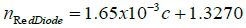(3)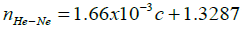(4)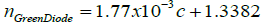(5)

The slope of the linear curve fitting and standard errors are in the range of (1.65-1.77) × 10-3 and (3.3840-1.375) × 10-5 respectively. The difference in the slope value indicated the dependence of refractive index on the wavelength of laser light.

Figure 2: Shows the refractive index versus concentrations of sugar solution using Red Diode, He-Ne and Green Diode laser in standard solutions.

A comparison of our experimental results with the available literature data indicated that the results are quite similar with those previously reported by other authors [2-4]. In Table the last two columns shows the refractive index of sucrose solutions reported using Abbe refractometer  and Sodium lamp at the wavelength (589.29 nm) . As indicated in Table 1 our data and the previously reported results using different techniques are quite consistent with deviation of the order of (10-3). Figure 3 displays the residual of the RI at different concentration of sugar solutions. It shows the good linear relationship between the measured RI and measured RI and concentration.

Concentration (%) RI values Using RedDiode RI values Using  He-Ne RI values Using Green Diode Literatures value  Literatures value 
0 1.3264 1.3282 1.3376 1.3360
5 1.3357 1.3380 1.3467 1.3412 1.3450
10 1.3448 1.3465 1.3557 1.3481
15 1.3532 1.3558 1.3655 1.3553
20 1.3584 1.3602 1.3742 1.3623 1.3650
25 1.3668 1.3688 1.3830 1.3775
30 1.3741 1.3770 1.3916
35 1.3818 1.3848 1.3991
40 1.3918 1.3948 1.4078 1.3947 1.3950
45 1.4018 1.4041 1.4174
50 1.4117 1.4140 1.4272 1.4119

Table 1: Comparison of refractive index of sugar solutions of our results using different laser light (532, 632.8 and 650 nm) and the reported data using sodium lamp (589.29 nm) and Abbe refractometer.

Effects of wavelength on the refractive index

Dispersion of light measures the change of the refractive index with wavelength. It can be explained by applying electromagnetic theory to the molecular structure of the materials. If an electromagnetic wave impinges on an atom or molecule the bound charge vibrate at the frequency of the incident wave. The bound charges have resonance frequency at certain wavelength. Figure 4 shows the refractive index versus wavelength of sugar solution (30%) at room temperature. The dots are experimental data and solid lines are Cauchy’s equation fitted to the experimental data. Over the visible wavelength regions, the Cauchy’s equation quite fitted to the experimentally measured refractive indices. The three Cauchy fitting parameters extracted are: A=1.38399, B=0.01611 μm2, C=0.00516 μm4 and the correlation coefficient (R2=0.97) respectively. The obtained fitting parameters are similar with the previously reported refractive indices of liquid crystals . The three Cauchy coefficients are the peculiar properties of the materials.

Figure 4: Wavelength dependent refractive index for sugar solution of 30% at room temperature a. the dot indicate experimental results and b. the line indicate Cauchy’s Equation.

The empirical expression for the wavelength dependent refractive index of sugar solution (30%) obtained by fitting Cauchy’s equation to the experimental data using non-linear curve are given by the following Equation (7)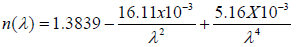(6)

Using eqn. (6), the calculated refractive index at the wavelength of 532 and 650 nm are 1.3915 and 1.3748 respectively.

Effects of temperature on refractive index of sugar solutions

Figure 5 illustrates the dependence of refractive index on temperatures investigated using laser lights with different sugar concentrations. It indicates that at the fixed concentration, the refractive index decrease linearly with the temperatures (308.15-340.15 K). From the fitting curve the slope increase as the concentration of the solution increases. The linear equations relate the refractive index with the temperature for 40% sugar solution can be expressed using eqns. (8) and (9).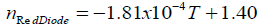(7)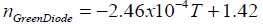(8)

Where, nRedDiode and nGreenDiode represents the refractive index obtained using Red and Green Diode laser respectively. The coefficients of the temperature deduced from the experimental data are the order of 10-4 for all lasers light. The temperature coefficient of RI for different concentrations can be used to calculate the thermal expansion coefficient .

Figure 5: The dependence of refractive index of sugar solution on temperature at different concentrations using a. Red diode laser (650 nm) and b. Green Diode laser (532 nm).

Variation of dn/dc with temperature

Figure 6 shows the variation of dn/dc vs. temperature. We can see that the derivative of refractive index with respect to concentration change highly with temperature and the depending coefficients are in the order of 10-7. The dn/dc of the solution decreases with the increases of the temperature. From fitting linear equation to experimental data the following empirical eqn. (9) obtained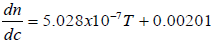(9)

Determination of sugar contents in soft drink beverages solutions

Equations (4-6) show the relation between the refractive index and concentration of sugar solutions using three lasers light. Using eqn. (4) the contents of sugar in soft drink beverages solutions (Pepsi, Coca, 7Up, Sprite, Fanta and Mirinda) were determined. In Table 2 the first column shows the average refractive index in different soft drinks measured using Red Diode laser (λ = 650nm).

Items Refractive index Sugar contents (%) or g/100ml Content of sugar soft drink beverages from other literatures
Pepsi 1.3473 12.30± 0.001 7-14  
9.91-13.55 .
8.20-11.10 
Coca 1.3472 12.24± 0.001
7Up 1.3472 12.24± 0.002
Sprite 1.3412 8.62± 0.001
Fanta 1.3416 8.85± 0.001
Mirinda 1.3463 11.70± 0.002

Table 2: The Refractive indices and sugar contents of soft drink beverages (Coca, Pepsi, Mirind, sprite, 7up and Fanta) and literatures report.

The values of sugar contents determined in soft drinks using this technique is within the range of 8.62-12.30% which is also quite similar with previously reported by other techniques such as densitometer and refractometer, Spectrophotometeric, and HPLC [19-21]. The assessment of the sugar level of the ten soft drinks samples studied using densitometer and refractometer is within the range of 7-14% . Also the sugar contents of soft drinks available in Nigeria studied by spectrophotometer are in the range of 9.91-13.55% . The sugar contents of the three soft drinks (Pepsi, Coca-cola and Sprite) studied using powerful instruments HPLC are 11.40, 11.10 and 8.20 respectively . From the study results the new technique analyzed the level of sugar within the acceptable limit of regulatory standard. Moreover, the techniques are also simple, fast and inexpensive.

#### Conclusions

We have investigated the concentration, temperature and wavelength dependent refractive index of sugar solution and an empirical expression established for the concentration and temperature coefficients of the solutions. By this method the contents of sugar in soft drink beverages determined and the results are quite similar with the previously reported literatures. These findings can help to improve the fundamental understanding of binary mixtures and significant for the determinations of the concentration. The techniques are also simple, fast and inexpensive.

#### References

Citation: Belay A, Assefa G (2018) Concentration, Wavelength and Temperature Dependent Refractive Index of Sugar Solutions and Methods of Determination Contents of Sugar in Soft Drink Beverages using Laser Lights. J Laser Opt Photonics 5: 187. DOI: 10.4172/2469-410X.1000187

Copyright: © 2018 Belay A, et al. This is an open-access article distributed under the terms of the Creative Commons Attribution License, which permits unrestricted use, distribution, and reproduction in any medium, provided the original author and source are credited.

Select your language of interest to view the total content in your interested language

##### Recommended Journals
Viewmore
###### Article Usage
• Total views: 14531
• [From(publication date): 0-2018 - Sep 22, 2019]
• Breakdown by view type
• HTML page views: 14208

## Post your commentCan't read the image? click here to refresh
###### Peer Reviewed Journals

Make the best use of Scientific Research and information from our 700 + peer reviewed, Open Access Journals

International Conferences 2019-20

Meet Inspiring Speakers and Experts at our 3000+ Global Annual Meetings

Top